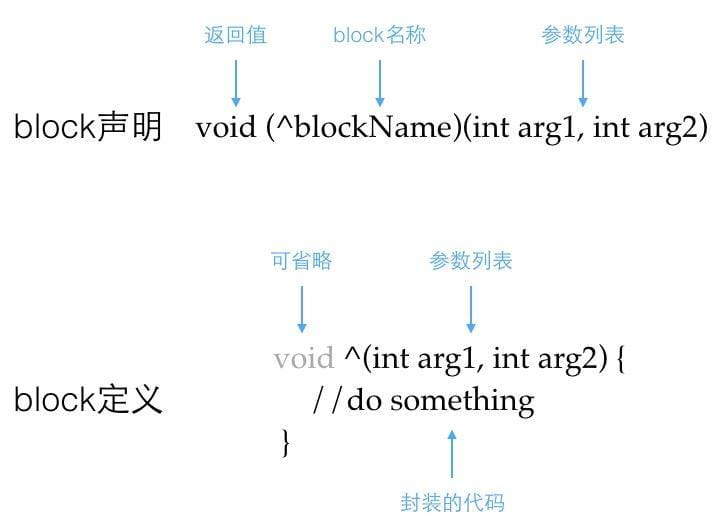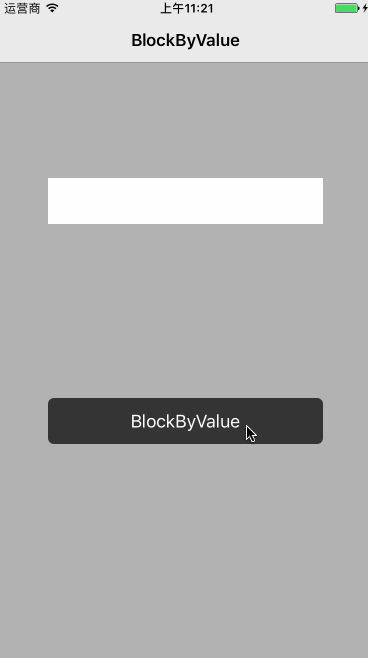### 关于 Block

``````bool executeSomeTask(void) {
//do something and return if success or not
}
bool (*taskPoint)(void);
taskPoint = something;
``````

1. block 的代码是内联的，效率高于函数调用
2. block 对于外部变量默认是只读属性
3. block 被 Objective-C 看成是对象处理

### Block 特性

#### 认识 Block

Block 代码结构如下：``````BOOL (^isInputEven)(int) = ^(int input) {
if (input % 2 == 0) {
return YES;
} else {
return NO;
}
};
``````

``````int x = -101;
NSLog(@"%d %@ number", x, isInputEven(x) ? @"is an even" : @"is not an even");
``````

#### Block 递归调用

Block 想要递归调用，代码块变量必须是全局变量或者是静态变量，这样在程序启动的时候 Block 变量就初始化了，可以递归调用。

``````static void (^ const blocks)(int) = ^(int i)
{
if (i > 0) {
NSLog(@"num:%d", i);
blocks(i - 1);
}
};
blocks(3);
``````

``````num:3
num:2
num:1
``````

#### 在 Block 中使用局部变量和全局变量

``````int global = 1000;

int main(int argc, const char * argv[])
{
@autoreleasepool {
void(^block)(void) = ^(void)
{
global++;
NSLog(@"global:%d", global);
};
block();
NSLog(@"global:%d", global);
}
return 0;
}
``````

``````global:1001
global:1001
``````

``````int local = 500;

void(^block)(void) = ^(void)
{
local++;
NSLog(@"local:%d", local);
};
block();
NSLog(@"local:%d", local);
``````

``````__block int local = 500;
void(^block)(void) = ^(void)
{
local++;
NSLog(@"local:%d", local);
};
block();
NSLog(@"local:%d", local);
``````

``````local:501
local:501
``````

#### Block 回调

``````#import <UIKit/UIKit.h>

typedef void (^BlockValue)(NSString *value);

@interface SecViewController : UIViewController

@property (copy, nonatomic) BlockValue myBlock;

@end
``````

``````-(void)btnClicked{
self.myBlock(self.textField.text);
[self dismissViewControllerAnimated:YES completion:nil];
}
``````

``````-(void)btnClicked{

SecViewController *sec = [[SecViewController alloc]init];
// 弱引用转换,为了防止循环引用
__weak RootViewController *weakSelf = self;
sec.myBlock = ^(NSString *value) {
weakSelf.label.text = value;
};
[self presentViewController:sec animated:YES completion:nil];
}
``````